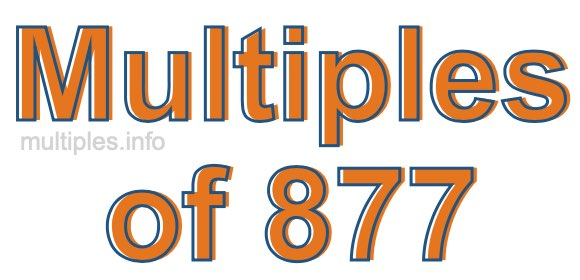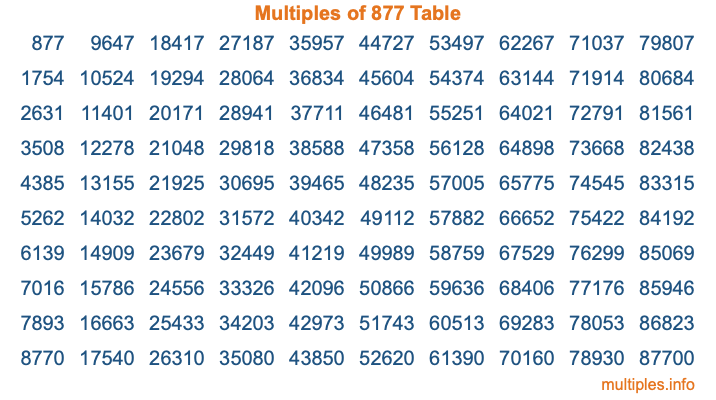Multiples of 877Welcome to the Multiples of 877 page. Here we will first teach you everything you will ever need to know about the multiples of 877, and then give you a study guide summary of everything we taught you to make sure you remember it all. Use this page to look up facts and learn information about the multiples of 877. This page will make you a multiples of eight hundred seventy-seven expert!

Definition of Multiples of 877
Multiples of 877 are all the numbers that when divided by 877 equal an integer. Each of the multiples of 877 are called a multiple. A multiple of 877 is created by multiplying 877 by an integer.

Therefore, to create a list of multiples of 877, you start with 1 multiplied by 877, then 2 multiplied by 877, then 3 multiplied by 877, and so on for as long as you want. Thus, the list of the first five multiples of 877 is 877, 1754, 2631, 3508, and 4385. To see a larger list of multiples of 877, see the printable image of Multiples of 877 further down on this page. We also have a category where you can choose any nth multiple of 877.

Multiples of 877 Checker
The Multiples of 877 Checker below checks to see if any number of your choice is a multiple of 877. In other words, it checks to see if there is any number (integer) that when multiplied by 877 will equal your number. To do that, we divide your number by 877. If the the quotient is an integer, then your number is a multiple of 877.

Is  a multiple of 877?

Least Common Multiple of 877 and ...
A Least Common Multiple (LCM) is the lowest multiple that two or more numbers have in common. This is also called the smallest common multiple or lowest common multiple and is useful to know when you are adding our subtracting fractions. Enter one or more numbers below (877 is already entered) to find the LCM.

Check out our LCM Calculator if you need more details about the Least Common Multiple or if you need the LCM for different numbers for adding and subtraction fractions.

nth Multiple of 877
As we stated above, 877 is the first multiple of 877, 1754 is the second multiple of 877, 2631 is the third multiple of 877, and so on. Enter a number below to find the nth multiple of 877.

th multiple of 877

Multiples of 877 vs Factors of 877
877 is a multiple of 877 and a factor of 877, but that is where the similarities end. All postive multiples of 877 are 877 or greater than 877. All positive factors of 877 are 877 or less than 877.

Below is the beginning list of multiples of 877 and the factors of 877 so you can compare:

Multiples of 877: 877, 1754, 2631, 3508, 4385, etc.

Factors of 877: 1, 877

As you can see, the multiples of 877 are all the numbers that you can divide by 877 to get a whole number. The factors of 877, on the other hand, are all the whole numbers that you can multiply by another whole number to get 877.

It's also interesting to note that if a number (x) is a factor of 877, then 877 will also be a multiple of that number (x).

Multiples of 877 vs Divisors of 877
The divisors of 877 are all the integers that 877 can be divided by evenly. Below is a list of the divisors of 877.

Divisors of 877: 1, 877

The interesting thing to note here is that if you take any multiple of 877 and divide it by a divisor of 877, you will see that the quotient is an integer.

Multiples of 877 Table
Below is an image of the first 100 multiples of 877 in a table. The table is in chronological order, column by column. The first column has the first ten multiples of 877, the second column has the next ten multiples of 877, and so on.The Multiples of 877 Table is also referred to as the 877 Times Table or Times Table of 877. You are welcome to print out our table for your studies.

Negative Multiples of 877
Although not often discussed or needed in math, it is worth mentioning that you can make a list of negative multiples of 877 by multiplying 877 by -1, then by -2, then by -3, and so on, to get the following list of negative multiples of 877:

-877, -1754, -2631, -3508, -4385, etc.

Multiples of 877 Summary
Below is a summary of important Multiples of 877 facts that we have discussed on this page. To retain the knowledge on this page, we recommend that you read through the summary and explain to yourself or a study partner why they hold true.

There are an infinite number of multiples of 877.

A multiple of 877 divided by 877 will equal a whole number.

877 divided by a factor of 877 equals a divisor of 877.

The nth multiple of 877 is n times 877.

The largest factor of 877 is equal to the first positive multiple of 877.

877 is a multiple of every factor of 877.

877 is a multiple of 877.

A multiple of 877 divided by a divisor of 877 equals an integer.

877 divided by a divisor of 877 equals a factor of 877.

Any integer times 877 will equal a multiple of 877.

Multiples of a Number
Here you can get the multiples of another number, all with the same attention to detail as we did for multiples of 877 on this page.

Multiples of
Multiples of 878
Did you find our page about multiples of eight hundred seventy-seven educational? Do you want more knowledge? Check out the multiples of the next number on our list!